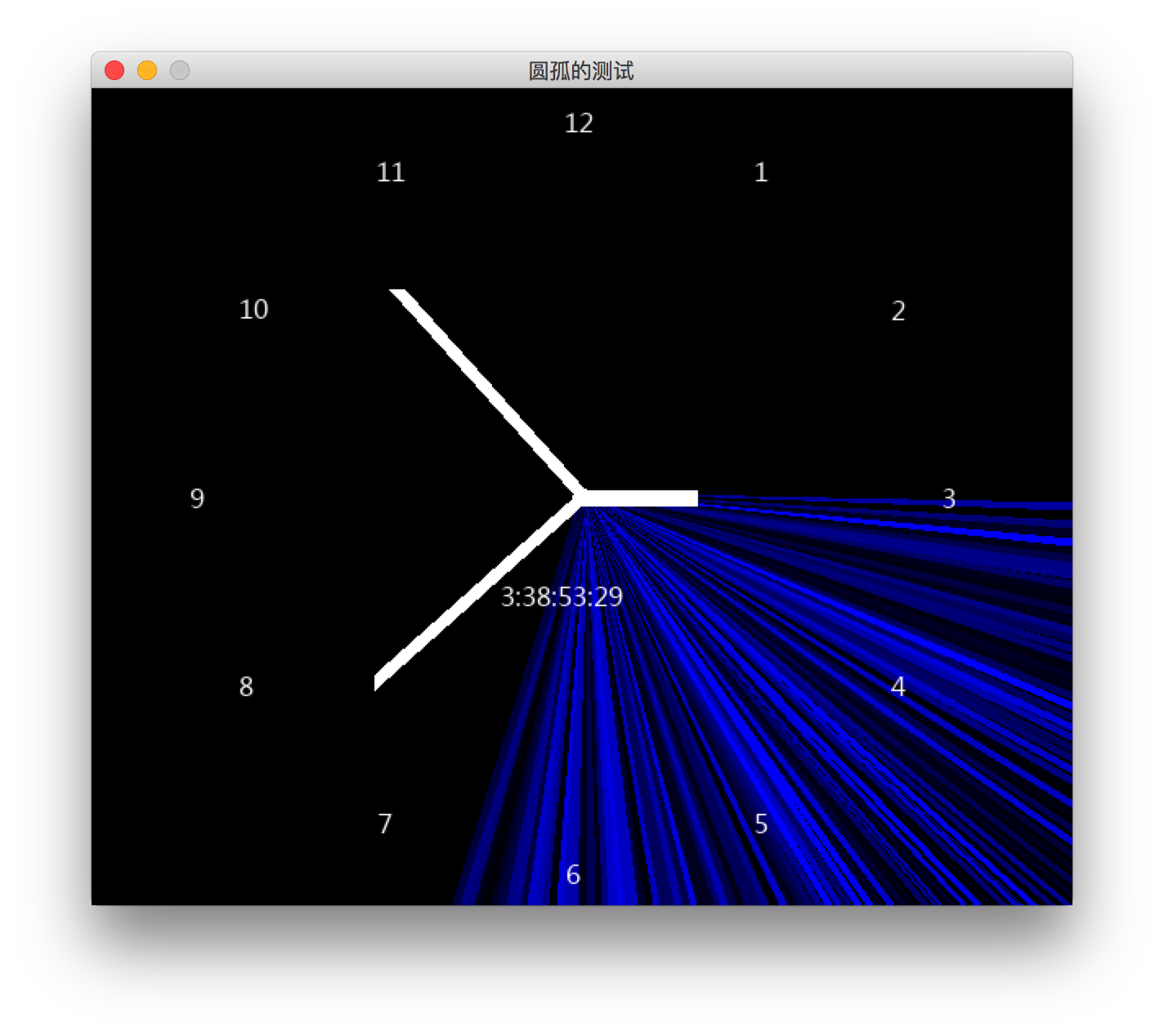# “编学编玩”用Pygame编写游戏（5）pygame绘制一个钟表

## 一些知识点

• diameter 圆的直径
• angle 角度
• 一个圆的完整的弧度 PI*2=6.28
• 1弧度的角度值为 360/6.28=57.3248
• 1度的弧度值为 6.28/360=0.0174
• math.degrees(x)将角度x从弧度转换为度。
• math.sin(x)返回x弧度的正弦值。
• math.cos(x)返回x弧度的余弦值。

## 圆周上点的坐标

``````x = math.cos(math.radians(angle)) * (radius)
``````

## 实例